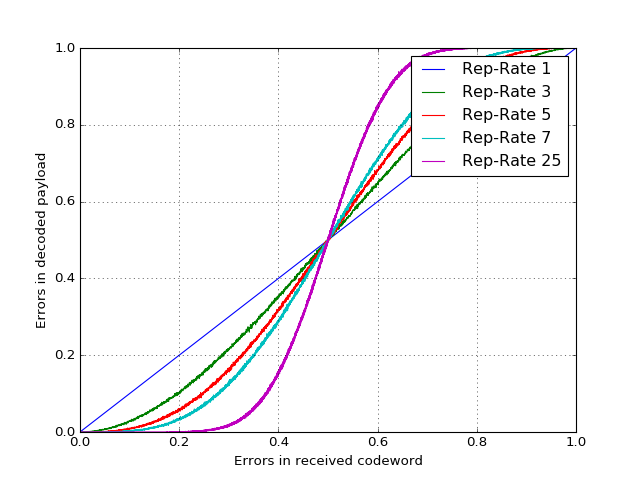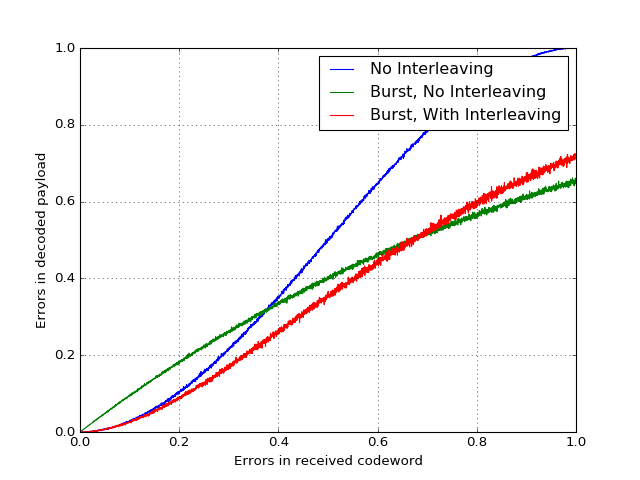# Hands-on Digital transmission with your soundcard¶

## 06 - Channel Coding, Frame Structure and Higher Layers (1)¶

In the previous notebook we eventually obtained a stable stream of bits that represented the actually transmitted bits. However, we pointed out that just a bit stream is not sufficient to transmit real information. One needs to know, what each bit means. That's why digital communication systems employ a frame structure, where depending on the position of the bit is has different meanings.

Moreover, due to noise or imperfect receiver algorithms like timing recovery, it is not guaranteed that every bit is transmitted correctly. Therefore, a so-called channel code or forward error correction (FEC) is applied to the data. These techniques add redundancy to the transmitted data such that the erroneous detection of some bits can be recovered at the receiver side.

In addition, it is important to know at the receiver, if a frame was detected correctly. To do that, checksums are applied to the data which needs to match the received data. If the checksum is wrong, most probably at least one bit in the frame is wrong and in the simplest case the frame will be discarded.

In the following notebooks, we will employ above techniques in a rudimentary fashion such that we will eventually be able to really transmit data from the transmitter to the receiver. This notebook focuses on channel coding, where we apply the most basic of all codes: repetition codes.

In :
import matplotlib
import matplotlib.pyplot as plt
%matplotlib notebook

import numpy as np
import sys; sys.path.append('..')

from collections import defaultdict

In :
%matplotlib notebook


### Channel coding¶

The purpose of channel coding (or forward error correction (FEC)) is to recover from bit errors that will inevitably occur during the transmission. These bit errors stem from thermal noise at the receiver, interference from other systems, synchronization errors, bad channel conditions and more. The idea of channel coding is to add redundancy to the transmitted bits, such that some bits can become erroneous but would still be corrected after decoding. Here, the coding rate $r$ is a central measure, which denotes the ratio between the number of transmitted bits and number the actually useful bits within this transmission.

Channel coding as a research topic is still very active with ongoing standardization of new generations of wirend and wireless systems. In this notebook, we will develop the most basic channel code (also the worst of them), the repetition code.

The idea of a repetition code is to repeat each bit several times at the transmitter. At the receiver, a majority decision is performed to decide if the bit was 1 or 0. In order to not run into a tie at the decoder, we require the number of repeated bits to be odd, such that there will always be a majority for either 1 or 0.

Let's write the simple encode function, taking the bits to be encoded and the number of repetitions:

In :
def repetition_encode(bits, inverseRate):
assert inverseRate % 2 == 1
rep = np.tile(bits, (inverseRate,1))
return rep.flatten(order='F')


Let's try this out:

In :
payload = np.array([1, 0, 1, 0, 1])
print ("Encoded (%02d bits): %s" % (len(encoded), encoded))

Payload ( 5 bits): [1     0     1     0     1]
Encoded (15 bits): [1 1 1 0 0 0 1 1 1 0 0 0 1 1 1]


As we see, each transmitted bits is repeated three times at the encoder output. Now, let's implement the simple majority decision decoder, and try it with the encoded bits from above:

In :
# Source code has been redacted in online version
# Omitting 7 lines of source code

In :
decoded = repetition_decode(encoded, 3)
print ("Decoded data (%2d bits): %s" % (len(decoded), decoded))

     Payload ( 5 bits): [1 0 1 0 1]
Decoded data ( 5 bits): [1 0 1 0 1]


Apparently, the decoding works. Now, let's try what happens, if we introduce some bit errors at the receiver and decode the data:

In :
def simulateErrors(payload, encoded, errorPositions):
received = encoded.copy(); received[errorPositions] ^= 1;  # flip the bits at errorPositions
print ("Transmitted: %s" % encoded)
print ("Errors     : [%s]" % " ".join('X' if i in errorPositions else ' ' for i in range(len(encoded))))

print ()
print ("Decoded    : %s" % decoded)

Transmitted: [1 1 1 0 0 0 1 1 1 0 0 0 1 1 1]
Received   : [1 1 1 0 0 1 1 1 0 0 1 0 1 1 1]
Errors     : [          X     X   X        ]

Payload    : [1 0 1 0 1]
Decoded    : [1 0 1 0 1]


Good, the channel code could recover from the introduced bit errors. Now, let's see what happens if errors occur at a different position:

In :
simulateErrors(payload, encoded, errorPositions=[1,2,3])

Transmitted: [1 1 1 0 0 0 1 1 1 0 0 0 1 1 1]
Received   : [1 0 0 1 0 0 1 1 1 0 0 0 1 1 1]
Errors     : [  X X X                      ]

Payload    : [1 0 1 0 1]
Decoded    : [0 0 1 0 1]


This time, the channel code could not recover from the errors, since two out of three bits of the first repetition were corrupted. Now, assuming the bit errors occur at random positions, let's simulate a bit error curve:

In :
def countErrors(payloadLen, errorCount, inverseRate):

# choose random error positions
errors = np.random.choice(np.arange(len(encoded)), size=errorCount, replace=False)

# flip bits at error positions

N = nBitsPayload # Number of bits in each frame
I = iterationsPerError   # Number of codewords per error count
errors = defaultdict(list)

for e in range(maxErrors):
for i in range(I):
errors[e].append(countErrors(N, e, inverseRate))
errorMean = [sum(errors[e])/(N*I) for e in range(maxErrors)]
return np.arange(maxErrors)/(inverseRate*N), errorMean

In :
# Attention: This cell can take quite some time to finish
plt.plot(*simulateBitErrors(1000, 10, 1000, 1), label='Rep-Rate 1')
plt.plot(*simulateBitErrors(1000, 10, 3000, 3), label='Rep-Rate 3')
plt.plot(*simulateBitErrors(1000, 10, 5000, 5), label='Rep-Rate 5')
plt.plot(*simulateBitErrors(1000, 10, 7000, 7), label='Rep-Rate 7')
plt.plot(*simulateBitErrors(1000, 10, 25000, 25), label='Rep-Rate 25')
plt.grid(True);
plt.legend();We plot the ratio of errors in the encoded data vs the ratio of errors in the decoded data for different repetition rates. For the repetition-rate-1 code (i.e. no repetition), the curve is clear: Every error in the encoded data becomes a bit error in the decoded codeword, hence the line is straight.

The codes with actual repetition have a more interesting shape: For few bit errors, they outperform the non-redundant rate-1 code. However, as soon as there happen to be more than 50% errors in the received codeword, the number of errors in the decoded word lies above the curve of the rate-1 code, hence making it actually worse. In addition, note that a rate 3-code requires 3 times the number of bits to be transmitted.

All in all, these figures show that the repetition code indeed is a not-so-powerful channel code. However, it suffices for our purposes where we do not expect too many bit errors (i.e. we operate in the lower-left area) and hence the repetition code can be useful. Looking at the curve, we make a tradeoff between overhead and performance and choose the rate-3 code for subsequent analysis.

### Bit Interleaving¶

Another important aspect is the distribution of bit errors. In the previous simulation we assumed the bit errors occur randomly distributed over the codeword. However, in particular channels it can happen that bit errors occur concentrated. For example, imagine the symbol timing gets messed up by some interference or timing jitter at the transmitter. In this case, all bits are likely wrong as long as the timing recovery was not done. We say, the channel introduces bit errors in bursts. As we have seen before, the repetition code is vulnerable to repeated bit errors. As soon as the majority of the coded bits belonging to one payload bit are wrong, the code will not recover.

In order to combat burst errors, we can employ a technique called Interleaving. Interleaving mixes the order of the coded bits at the transmitter. At the receiver, the mixing is reversed, yielding the originally transmitted sequence. However, through the demixing, error bursts that occured during transmission are distributed.

Let's implement the most simple interleaver, a so-called random interleaver. For a given codeword length we generate a random permutation and fix it for the entire transmission. We note that both the transmitter and the receiver need to have knowledge of this permutation:

In :
# Source code has been redacted in online version
# Omitting 6 lines of source code


For illustration purposes, let's use letters as transmit symbols and interleave and deinterleave them:

In :
# Source code has been redacted in online version
# Omitting 9 lines of source code

Counter      :  [0   1   2   3   4   5   6   7   8   9]
Codeword     : ['A' 'B' 'C' 'D' 'E' 'F' 'G' 'H' 'I' 'J']

Interleaver  :  [3   0   7   2   5   1   8   6   9   4]
Interleaved  : ['D' 'A' 'H' 'C' 'F' 'B' 'I' 'G' 'J' 'E']

Deinterleaver:  [1   5   3   0   9   4   7   2   6   8]
Deinterleaved: ['A' 'B' 'C' 'D' 'E' 'F' 'G' 'H' 'I' 'J']


As we see, the interleaver mixes the order of the transmit symbols and the deinterleaver puts them back into correct order. Now, let's run a bit error rate simulation when errors occur in bursts:

In :
# Source code has been redacted in online version
# Omitting 43 lines of source code

In :
plt.figure()
plt.plot(*simulateBitErrors(1000, 10, 3000, 3), label='No Interleaving')
plt.plot(*simulateBitErrorsWithBurst(1000, 10, 3000, 3, useInterleaving=False), label='Burst, No Interleaving')
plt.plot(*simulateBitErrorsWithBurst(1000, 10, 3000, 3, useInterleaving=True), label='Burst, With Interleaving')Looking at the curves, we see that if relatively few errors occur in the stream, interleaving brings down the bit error rate to the case when errors occur randomly and not in burst. Hence, we will use the interleaving technique in our transmission system. Note, that the results for more than roughly 50% errors for the burst case are not accurate. The burst creation does not consider that burst cannot overlap. Hence, with many errors it is likely, that burst overlap and therefore fewer errors occur effectively. You can clearly see this discrepancy in the graph, as the number of errors in the decoded payload should be 100% when the number of errors in the received codeword are 100%. However, as we expect relatively few errors, this inaccuracy is irrelevant to us.

### Summary¶

In this, rather theoretic, notebook we introduced the technique of channel coding with the example of the most basic code, a repetition code. The channel code helps correct inevitable bit errors that occur during transmission. In addition we introduced interleaving to combat burst of errors.

In the next notebook, we will introduce a rudimentary frame structure including header and checksum, which we will use in our transmission system.